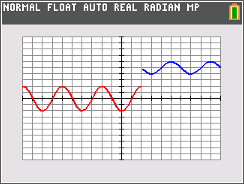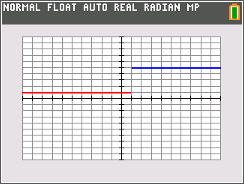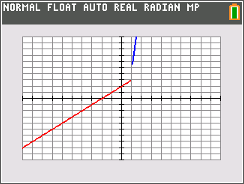••• ##### Device
• TI-84 Plus
• TI-84 Plus Silver Edition
•TI-84 Plus C Silver Edition
•TI-84 Plus CE

# Calculus: Making Limits Exist

by Texas Instruments#### Overview

In this activity, students will graph piecewise functions and evaluate numerically and graphically the left hand limit and the right hand limit of the function as x approaches a given number, c.

#### Key Steps

•Before changing the value of a, students will graphically estimate the limit of f(x) as x approaches 1 from the left and the right.

•Before changing the value of a, students will graphically estimate the limit of g(x) as x approaches 1 from the left and the right.

•Before changing the value of a, students will graphically estimate the limit of h(x) as x approaches 1 from the left and the right.